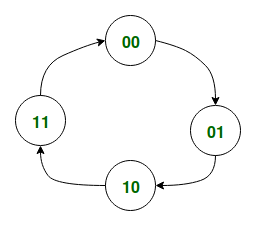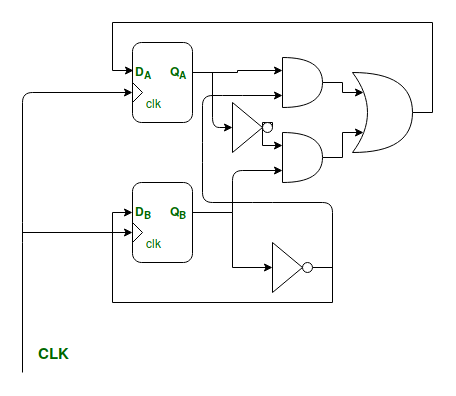# RTL (Register Transfer Level) design vs Sequential logic design

In this article we try to explain the fundamental differences between Register Transfer Level (RTL) Design and Sequential Logic Design.

In the RTL Design methodology different types of registers such as Counters, Shift Register, SIPO (Serial In Parallel Out), PISO (Parallel In Serial Out) are used as the basic building blocks for any Sequential Logic Circuits.

On the other hand Synchronous Sequential Logic Design methodology different logic gates and different memory elements such as flip flops (to store the state of circuit at any time) is used as the basic building blocks for sequential logic circuits.

The Synchronous Sequential Logic Design Process using state diagram and its shortcomings are explained in the following example:

Lets say, we are to design a 2-bit synchronous Binary Up Counter whose count sequence is:

00 -> 01 -> 10 -> 11 -> 00 -> 01 -> ..... so on.
1. Step-1: In the 1st step we draw a State Diagram representing the above sequential circuit.
The State Diagram representing the above counter is shown below:Figure – State Diagram for 2-bit UP Counter

2. Step-2: In the next step we derive the State Table from the above given State Diagram

The State Table is as given below:

Present State Q(n) Next State Q(n+1) Output
00 01 01
01 10 10
10 11 11
11 00 00

3. Step-3: In the third step we need to choose the type of flip flop we will be using to store the state of the circuit, for simplicity, we will be considering the Positive Edge Triggered D-type Flip-Flop.We also need to determine the number of Flip-Flops required to represent the internal state of the circuit. The general formula for the number of Flip-Flops required:
Total Number of Flip-Flops =Where,
N = Total Number of States in State Table   

Then we need to note down the Excitation Table for chosen Flip-Flop. The Excitation Table for the D-type Flip-Flop is shown below:

Present State Q(n) Next State Q(n+1) D
X 0 0
X 1 1

4. Step-4: In this step we combine the State Table from the 2nd step with the excitation table of the previous step as follows:0 0 0 1 0 1
0 1 1 0 1 0
1 0 1 1 1 1
1 1 0 0 0 0

5. Step-5: Next, from the above table we try to expressas boolean functions of.

In this case the expression for bothare trivial.6. The Final Sequential Circuit is shown below:Figure – The Final Circuit

Shortcomings of the above process:

• From the above example we observe that the Synchronous Sequential Logic Design process is a fairly involved process and requires us to go through a sequence of well defined steps even for simple circuits like the one above.
• Secondly, if the number of states become large then this process becomes cumbersome and time-consuming, and sometimes even impossible.

To Address the above drawbacks of the Sequential Logic Design process and to enable Digital Designers to design circuits of higher complexity with ease, the RTL design methodology was introduced. The most popular example of RTL Design is that of a Processor, which is nothing but a very sophisticated Finite State Machine with a very large number of states.

The main differences between RTL Design and Sequential Logic Design are summarized below:

RTL Design Sequential Logic Design
In RTL Design the basic building blocks are registers, Multiplexers, Adders. In Sequential Logic Design the basic building blocks are the Logic Gates, Flip-Flops.
RTL Design is much closer to the Behavioural Design of a Logic Circuit as it models the data flow among different registers, and hence is much more intuitive. Sequential Logic Design process is more mechanical in nature as compared to RTL design process
Finally, RTL Modelling allows us to synthesize complex circuits with a large number of States with much more ease as compared to Sequential Logic Design. The Sequential Logic Design techniques are only applicable to circuits having a small number of states.

Attention reader! Don’t stop learning now. Get hold of all the important CS Theory concepts for SDE interviews with the CS Theory Course at a student-friendly price and become industry ready.

My Personal Notes arrow_drop_upCheck out this Author's contributed articles.

If you like GeeksforGeeks and would like to contribute, you can also write an article using contribute.geeksforgeeks.org or mail your article to contribute@geeksforgeeks.org. See your article appearing on the GeeksforGeeks main page and help other Geeks.

Please Improve this article if you find anything incorrect by clicking on the "Improve Article" button below.